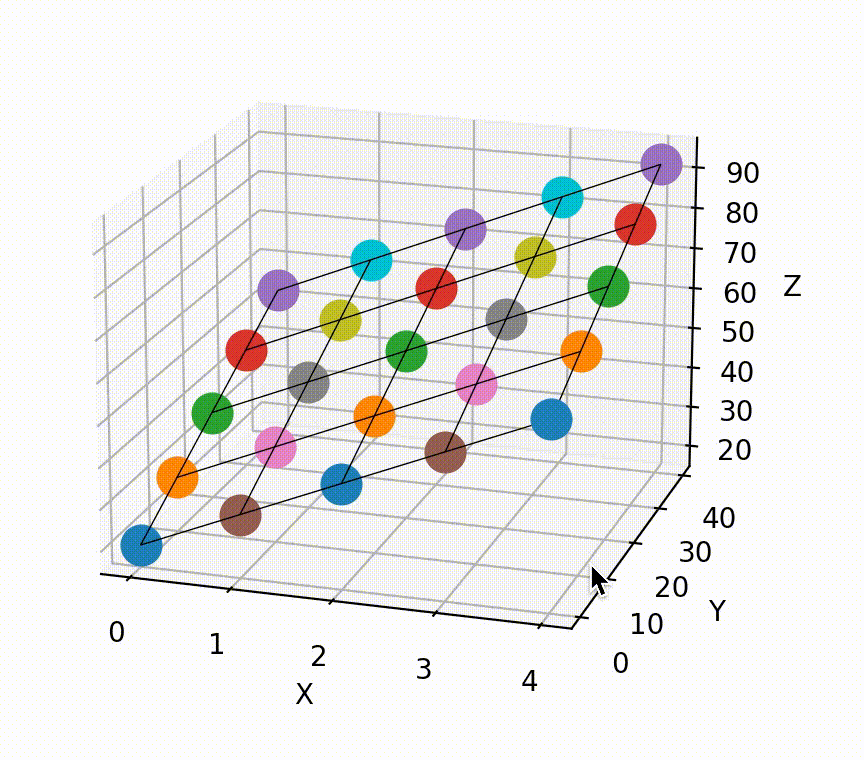# Matplotlib 3d scatterplot with wireframe plane example.

Here you see an example of a scatterplot with two inputs and one output. A regression plane is drawn with a wireframe.How can you re-create this?

Pip-install matplotlib and numpy

`pip install matplotlib`
Matplotlib also installs numpy automatically.

Copy and paste the code.

```import matplotlib.pyplot as plt
import numpy as np

def predict(x, y):
return x * 10 + y * 0.8 + 20

x_axis = [0, 1, 2, 3, 4]
y_axis = [0, 10, 20, 30, 40]

X, Y = np.meshgrid(x_axis, y_axis)
Z = predict(X, Y)

fig = plt.figure()